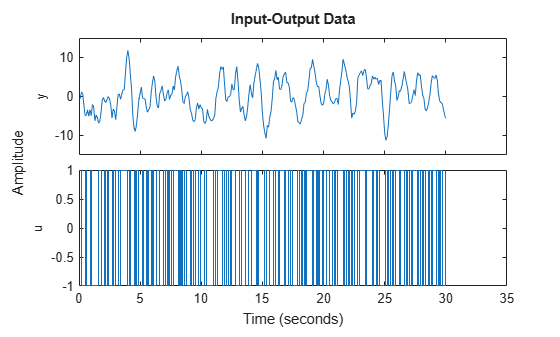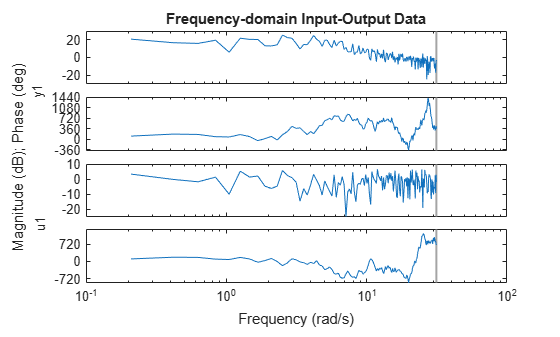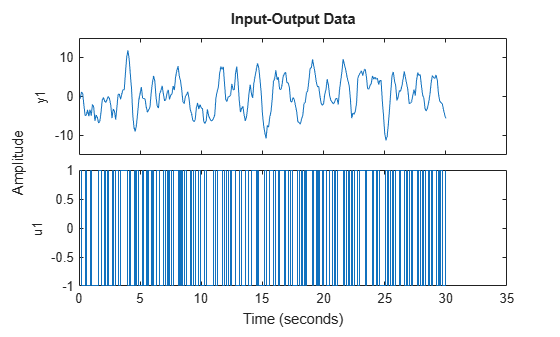Documentation

iddata/plot

Plot input-output data

Description

example

plot(data) plots the input and output channels of an iddata object. The outputs are plotted on the top axes and the inputs on the bottom axes.

• For time-domain data, the input and output signals are plotted as a function of time. Depending on the InterSample property of the iddata object, the input signals are plotted as linearly interpolated curves or as staircase plots. For example, if data.InterSample = 'zoh', the input is piecewise constant between sampling points, and it is then plotted accordingly.

• For frequency-domain data, the magnitude and phase of each input and output signal is plotted over the available frequency span.

To plot a subselection of the data, use subreferencing:

• plot(data(201:300)) plots the samples 201 to 300 in the dataset data.

• plot(data(201:300,'Altitude',{'Angle_of_attack','Speed'})) plots the chosen samples of output named Altitude and inputs named Angle_of_attack and Speed.

• plot(data(:,[3 4],[3:7])) plots all samples of output channel numbers 3 and 4 and input numbers 3 through 7.

plot(data,LineSpec) specifies the color, line style and marker symbol for the dataset.

plot(data1,...,dataN) plots multiple datasets. The number of plot axes are determined by the number of unique input and output names among all the datasets.

example

plot(data1,LineSpec1...,dataN,LineSpecN) specifies the line style, marker type, and color for each dataset. You can mix data,LineSpec pairs with data. For example, plot(data1,data2,LineSpec2,data3).

example

plot(axes_handle,___) plots into the axes with handle axes_handle instead of into the current axes (gca). This syntax can include any of the input argument combinations in the previous syntaxes.

example

plot(___,plotoptions) specifies the plot options. This syntax can include any of the input argument combinations in the previous syntaxes.

example

h = plot(___) returns the handle to the plot. You can use this handle to customize the plot with getoptions and setoptions.

Input Arguments

collapse all

Input-output data, specified as an iddata object. The data can be time-domain or frequency-domain. It can be a single- or multi-channel data, or single- or multi-experiment data.

Line style, marker symbol, and color, specified as a character vector. LineSpec takes values such as 'b', 'b+:'. For more information, see the plot reference page in the MATLAB® documentation.

Data Types: char

Axes handle, which is the reference to an axes object. Use the gca function to get the handle to the current axes, for example, axes_handle= gca.

Plot options, specified as an option set created using iddataPlotOptions.

Output Arguments

collapse all

Lineseries handle, returned as a scalar or vector. These are unique identifiers, which you can use to query and modify properties of a specific plot.

Examples

collapse all

Plot the data.

plot(z1)The output is plotted on the top axes and the input on the bottom axes.

You can right-click the plot to explore its characteristics such as peak and mean values.

Convert the data to frequency domain.

zf = fft(z1);

Plot the data.

plot(zf);Generate input data.

u = idinput([100 1 20],'sine',[],[],[5 10 1]);
u = iddata([],u,1,'per',100);

Generate output data.

sys = idtf(1,[1 2 1]);
y = sim(sys,u);

Plot only the input.

plot(u)Plot only the output.

plot(y)Plot the input and output together.

plot(y,u)Alternatively, you can use plot(iddata(y,u)) .

Create a multi-experiment data set.

zm = merge(z1,z2);

Plot the data.

plot(zm);For multi-experiment data, each experiment is treated as a separate data set. You can right-click the plots to view their characteristics.

Specify the linestyle properties.

plot(z1,'y:*',z2,'b')Create a figure with two subplots and return the handle to each subplot axes, s(1) and s(2).

figure; % new figure
s(1) = subplot(1,2,1); % left subplot
s(2) = subplot(1,2,2); % right subplotLoad the data sets.

Create a data plot in each axes referring to the axes handles.

plot(s(1),z1)
plot(s(2),z2)Configure a time plot.

opt = iddataPlotOptions('time');

View the plot in minutes time units.

opt.TimeUnits = 'minutes';

Turn grid on.

opt.Grid = 'on';

Create plot with the options specified by opt.

plot(z1, opt);Create a data plot and return the handle.

h = plot(z1);Set the time unit on the plot.

setoptions(h,'TimeUnits','minutes');Generate data with two inputs and one output.

z = iddata(randn(100,1),rand(100,2));

Configure a time plot.

opt = iddataPlotOptions('time');

Plot the data.

h = plot(z,opt);Change the orientation of the plots such that all inputs are plotted in one column, and all outputs are in a second column.

opt.Orientation = 'two-column';
h = plot(z,opt);Alternatively, use setoptions.

setoptions(h,'Orientation','two-column')

You can also change the orientation by right-clicking the plot and choosing Orientation in the context menu.

Tips

• Right-clicking the plot opens the context menu where you can access the following options and plot controls:

• Datasets — View the datasets used in the plot.

• Characteristics — View data characteristics.

• Peak Value — Peak value of the data. Useful for transient data.

• Mean Value — Mean value of the data. Useful for steady-state data.

• Orientation — Choose orientation of the input and output plots.

• Output row and input row — (For datasets containing more than one input or output channel) Plot all outputs in one row and all inputs in a second row.

• Output column and input column — (For datasets containing more than one input or output channel) Plot all outputs in one column and all inputs in a second column.

• Single row — Plot all inputs and outputs in one row.

• Single column— Plot all inputs and outputs in one column.

• I/O Grouping — (For datasets containing more than one input or output channel) Group input and output channels on the plot.

• I/O Selector — (For datasets containing more than one input or output channel) Select a subset of the input and output channels to plot. By default, all input and output channels are plotted.

• Grid — Add grids to your plot.

• Normalize — Normalize the y-scale of all data in the plot.

• Properties — Open the Property Editor dialog box, where you can customize plot attributes.